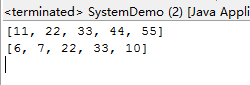# JAVA基础再回首（十二）——Character类、Math类、Random类、System类

### 最可怕的是，那些富二代比你还努力啊

• 自怨自艾是世界上最傻逼最不酷的事了。
你苦苦追求的上限，不过是人家的起点。
那你先到上限再说啊！
你觉得人家是富二代，你再怎么努力都超不过了？
那你先把那些不是富二代的大多数超过了再说呀。
你都不是富二代了，你都输在起跑线上了，所以你更要拼尽全力啊。
正是因为没有爹可以拼，我们才要拼命啊。

## Character类

• Character类概述
• Character 类在对象中包装一个基本类型 char 的值，此外，该类提供了几种方法，以确定字符的类别（小写字母，数字，等等），并将字符从大写转换成小写，反之亦然
• 构造方法
• public Character(char value)
public class CharacterDemo {
public static void main(String[] args) {
// 创建对象
//       Character ch = new Character((char) 97);
Character ch = new Character('a');
System.out.println("ch:" + ch);
//运行程序后可以发现：这两个对象的结果是相同的
}
}
• Character类成员方法
• public static boolean isUpperCase(char ch):判断给定的字符是否是大写字符
• public static boolean isLowerCase(char ch):判断给定的字符是否是小写字符
• public static boolean isDigit(char ch):判断给定的字符是否是数字字符
• public static char toUpperCase(char ch):把给定的字符转换为大写字符
• public static char toLowerCase(char ch):把给定的字符转换为小写字符

public class CharacterDemo {
public static void main(String[] args) {
// public static boolean isUpperCase(char ch):判断给定的字符是否是大写字符
System.out.println("isUpperCase:" + Character.isUpperCase('A'));
System.out.println("isUpperCase:" + Character.isUpperCase('a'));
System.out.println("isUpperCase:" + Character.isUpperCase('0'));

// public static boolean isLowerCase(char ch):判断给定的字符是否是小写字符
System.out.println("isLowerCase:" + Character.isLowerCase('A'));
System.out.println("isLowerCase:" + Character.isLowerCase('a'));
System.out.println("isLowerCase:" + Character.isLowerCase('0'));

// public static boolean isDigit(char ch):判断给定的字符是否是数字字符
System.out.println("isDigit:" + Character.isDigit('A'));
System.out.println("isDigit:" + Character.isDigit('a'));
System.out.println("isDigit:" + Character.isDigit('0'));

// public static char toUpperCase(char ch):把给定的字符转换为大写字符
System.out.println("toUpperCase:" + Character.toUpperCase('A'));
System.out.println("toUpperCase:" + Character.toUpperCase('a'));

// public static char toLowerCase(char ch):把给定的字符转换为小写字符
System.out.println("toLowerCase:" + Character.toLowerCase('A'));
System.out.println("toLowerCase:" + Character.toLowerCase('a'));
}
}
//结果就不必多说了，很容易理解的。

//我们要做一个统计一个字符串中大写字母字符，小写字母字符，数字字符出现的次数。（比如"Adu123Messi"这个字符串）
public class CharacterTest {
public static void main(String[] args) {
//首先定义一个字符串
String str = "Adu123Messi";
//然后让我们定义三个统计变量
int bigCount = 0;//大写字母
int smallCount = 0;//小写字母
int numberCount = 0;//数字
// 把字符串转换为字符数组。
char[] chs = str.toCharArray();

// 遍历字符数组获取到每一个字符
for (int x = 0; x < chs.length; x++) {
char ch = chs[x];

// 判断该字符
if (Character.isUpperCase(ch)) {
bigCount++;
} else if (Character.isLowerCase(ch)) {
smallCount++;
} else if (Character.isDigit(ch)) {
numberCount++;
}
}
// 输出结果
System.out.println("大写字母：" + bigCount + "个");
System.out.println("小写字母：" + smallCount + "个");
System.out.println("数字字符：" + numberCount + "个");
}
}


## Math类

• Math类概述
• Math 类包含用于执行基本数学运算的方法，如初等指数、对数、平方根和三角函数。
• 成员变量
• public static final double PI
• public static final double E
• 成员方法
• public static int abs(int a)：绝对值
• public static double ceil(double a):向上取整
• public static double floor(double a):向下取整
• public static int max(int a,int b):最大值
• public static double pow(double a,double b):a的b次幂
• public static double random():随机数 [0.0,1.0)
• public static int round(float a) 四舍五入
• public static double sqrt(double a):正平方根

public class MathDemo {
public static void main(String[] args) {
// public static final double PI
System.out.println("PI:" + Math.PI);//PI:3.141592653589793
// public static final double E
System.out.println("E:" + Math.E);//E:2.718281828459045

// public static int abs(int a)：绝对值
System.out.println("abs:" + Math.abs(10));
System.out.println("abs:" + Math.abs(-10));

// public static double ceil(double a):向上取整
System.out.println("ceil:" + Math.ceil(12.34));//13.0
System.out.println("ceil:" + Math.ceil(12.56));//13.0

// public static double floor(double a):向下取整
System.out.println("floor:" + Math.floor(12.34));//12.0
System.out.println("floor:" + Math.floor(12.56));//12.0

// public static int max(int a,int b):最大值
System.out.println("max:" + Math.max(12, 23));//23
// 获取三个数据中的最大值
// 方法的嵌套调用
System.out.println("max:" + Math.max(Math.max(12, 23), 18));
// 获取四个数据中的最大值
System.out.println("max:"+ Math.max(Math.max(12, 78), Math.max(34, 56)));

// public static double pow(double a,double b):a的b次幂
System.out.println("pow:" + Math.pow(2, 3));

// public static double random():随机数 [0.0,1.0)
System.out.println("random:" + Math.random());
// 获取一个1-100之间的随机数
System.out.println("random:" + ((int) (Math.random() * 100) + 1));

// public static int round(float a) 四舍五入(参数为double的自学)
System.out.println("round:" + Math.round(12.34f));//12
System.out.println("round:" + Math.round(12.56f));//13

//public static double sqrt(double a):正平方根
System.out.println("sqrt:"+Math.sqrt(4));
}
}

## Random类

• Random类概述
• 此类用于产生随机数
• 如果用相同的种子创建两个 Random 实例，则对每个实例进行相同的方法调用序列，它们将生成并返回相同的数字序列。
• 构造方法
• public Random()
• public Random(long seed)
• 成员方法
• public int nextInt()：返回的是int范围内的随机数
• public int nextInt(int n):返回的是[0,n)范围的内随机数
public class RandomDemo {
public static void main(String[] args) {
// 创建对象
Random r = new Random();
for (int x = 0; x < 10; x++) {
// int num = r.nextInt();//返回的是int范围内的随机数
int num = r.nextInt(100) + 1;//本来返回的是[0,100)范围的内随机数，+1后返回[1,100)
System.out.println(num);
}

//创建对象,给出指定的种子
Random r = new Random(123);
for (int x = 0; x < 10; x++) {
int num = r.nextInt(100) + 1;
System.out.println(num);//给定种子后，每次得到的随机数是相同的。
}
}
}

## System类

• System类概述
• System 类包含一些有用的类字段和方法。它不能被实例化。
• 成员方法
• public static void gc()：运行垃圾回收器。
• public static void exit(int status)：终止当前正在运行的 Java 虚拟机。参数用作状态码；根据惯例，非 0 的状态码表示异常终止。
• public static long currentTimeMillis()：返回以毫秒为单位的当前时间
• public static void arraycopy(Object src,int srcPos,Object dest,int destPos,int length)：从指定源数组中复制一个数组，复制从指定的位置开始，到目标数组的指定位置结束。

System.gc()可用于垃圾回收。

public class SystemDemo {
public static void main(String[] args) {
System.out.println("1945年的今天");
System.exit(0);
System.out.println("日本宣布无条件投降");
}
}
//运行结果可以看到控制台只打印了：1945年的今天
//因为System.exit(0);终止当前正在运行的 Java 虚拟机。

//单独使用这样目前对我们来说意义不大，所以我们可以统计下面这段程序的运行时间
long start = System.currentTimeMillis();
for (int x = 0; x < 100000; x++) {
System.out.println("hello" + x);
}
long end = System.currentTimeMillis();
System.out.println("共耗时：" + (end - start) + "毫秒");

src——源数组
srcPos——原数组中的起始位置
dest——目标数组
destPos——目标数组中的起始位置
length——要复制的数组元素的数量

public class SystemDemo {
public static void main(String[] args) {
// 定义数组
int[] arr = { 11, 22, 33, 44, 55 };
int[] arr2 = { 6, 7, 8, 9, 10 };

System.arraycopy(arr, 1, arr2, 2, 2);

System.out.println(Arrays.toString(arr));
System.out.println(Arrays.toString(arr2));
}
}